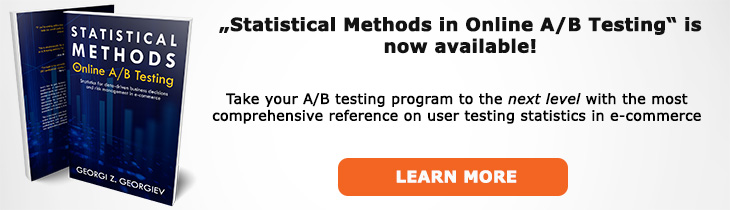# What does "Identically Distributed" mean?

Definition of Identically Distributed in the context of A/B testing (online controlled experiments).

## What is Identically Distributed?

Aliases: ID

Two variables (X,Y) are identically distributed (ID) if they have the same probability distribution. A sufficient condition for this is that CDF(X)=CDF(Y) where CDF stands for Cumulative Distribution Function. A textbook way of describing this would be to write P(x ≤ X) = P(y ≤ Y). Even more formally and in the general case of k random variables (X1, X2... Xn) it can be said that they are Identically Distributed if their marginal distributions have the same form: fk(Xkk)fk(Xk;θ), for all k=1,2...,n.

The necessity for variables to be identically distributed plays a significant role in a classical Null Hypothesis Statistical Test where the full definition of the null hypothesis states, among other things that the two variables are identically distributed.

An immediate consequence of the requirement for identical distributions is that data analyzed under the assumption of ID should have constant mean and variance. If the data has a trend this assumptions is violated and a statistical model with the ID assumption in it becomes misspecified.

## Related A/B Testing terms

Independent Variable

Independent Observation

Like this glossary entry? For an in-depth and comprehensive reading on A/B testing stats, check out the book "Statistical Methods in Online A/B Testing" by the author of this glossary, Georgi Georgiev.## Glossary Index by Letter

Select a letter to see all A/B testing terms starting with that letter or visit the Glossary homepage to see all.